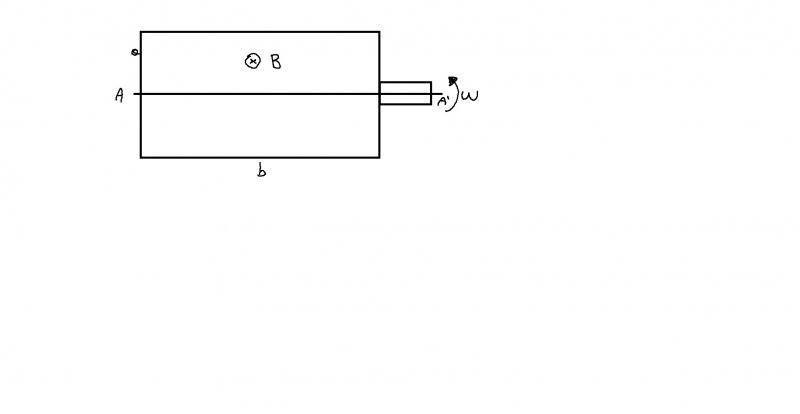# Faraday's and Lenz's law application

## Homework Statement

A rectangular coil with a and b sides can rotate around the axis AA' with angular velocity ω. It is in a magnetic field as in figure.Calculate the flux of B when the coil is orthogonal to the axis AA'. Calculate the $\epsilon_{max}$ and express the position of the coil.

## Homework Equations

$\epsilon = -\frac{d}{dt} \phi_B$

## The Attempt at a Solution

The flux when the coil is orthogonal to the axis AA' is 0.

$\phi_B = NBabcos\theta$

$\theta$ is 90°, so the flux is 0.

Now I calculate the emf.
$\epsilon = -\frac{d}{dt} \phi_B = NBab\omega sin(\omega t)$

The emf is max when $sin(\theta)$ is 1, so when $\theta$ is 90°.

Is it correct my procedure?
If yes, why emf is maximum when flux is 0?

Doc Al
Mentor
Calculate the flux of B when the coil is orthogonal to the axis AA'.
Since the coil rotates about that axis, it is always orthogonal to it. I assume you mean when the plane of the coil is perpendicular to the surface of the drawing.

Is it correct my procedure?
Yes.

If yes, why emf is maximum when flux is 0?
What matters is not where the emf is maximum but where its rate of change is maximum.

Edit: Oops... I meant flux, not emf, of course.

Last edited:
Since the coil rotates about that axis, it is always orthogonal to it. I assume you mean when the plane of the coil is perpendicular to the surface of the drawing.

Yes, sorry, I meant that.
Thank you!